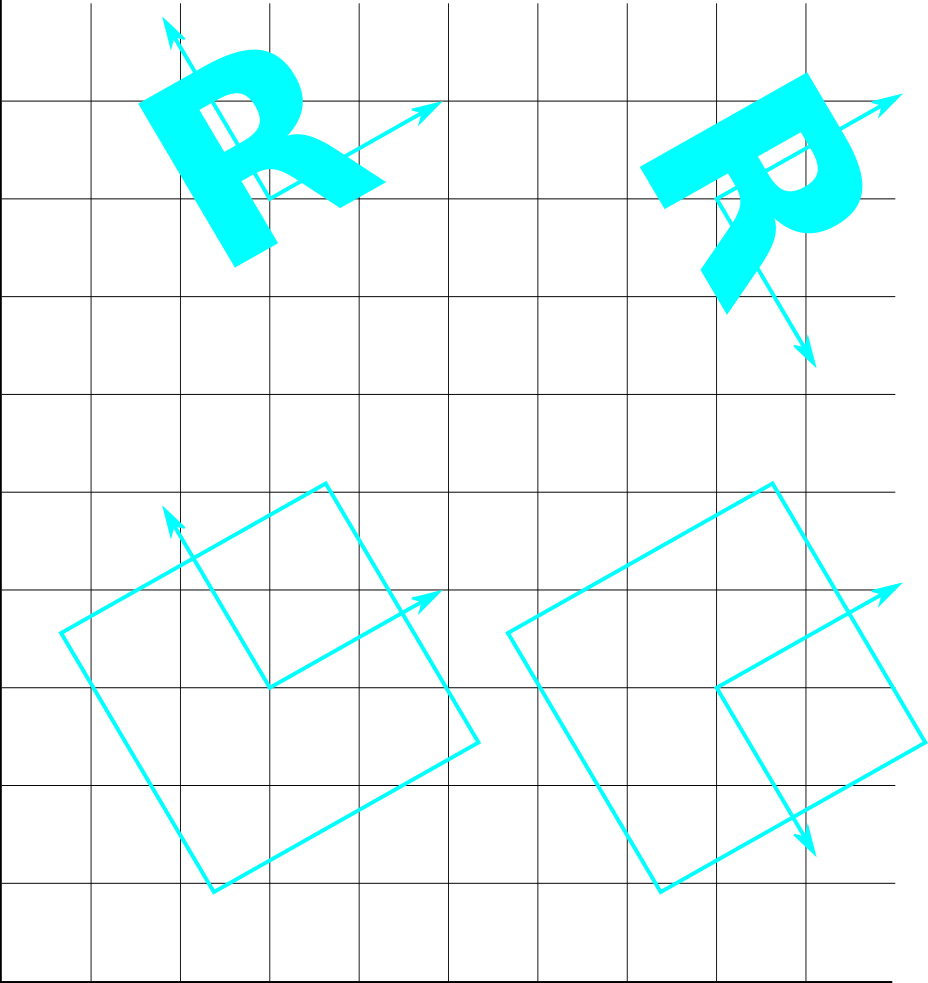# Orientation#

class orix.quaternion.Orientation(data: , symmetry: = None)[source]#

Bases: Misorientation

Orientations represent misorientations away from a reference of identity and have only one associated symmetry.

Orientations support binary subtraction, producing a misorientation. That is, to compute the misorientation from $$g_1$$ to $$g_2$$, call g_2 - g_1.

In orix, orientations and misorientations are distinguished from rotations only by the inclusion of a notion of symmetry. Consider the following example:Both objects have undergone the same rotations with respect to the reference. However, because the square has four-fold symmetry, it is indistinguishable in both cases, and hence has the same orientation.

Attributes

 Orientation.symmetry Symmetry. Orientation.unit Unit orientations.

Methods

 Orientation.angle_with(other[, degrees]) Return the smallest symmetry reduced angles of rotation transforming the orientations to the other orientations. Orientation.angle_with_outer(other[, lazy, ...]) Return the symmetry reduced smallest angle of rotation transforming every orientation in this instance to every orientation in another instance. Orientation.dot(other) Return the symmetry reduced dot products of the orientations and the other orientations. Return the symmetry reduced dot products of all orientations to all other orientations. Orientation.from_align_vectors(other, initial) Return an estimated orientation to optimally align vectors in the crystal and sample reference frames. Orientation.from_axes_angles(axes, angles[, ...]) Initialize from axis-angle pair(s). Orientation.from_euler(euler[, symmetry, ...]) Initialize from an array of Euler angles. Orientation.from_matrix(matrix[, symmetry]) Return orientation(s) from orientation matrices . Orientation.from_neo_euler(neo_euler[, symmetry]) Return orientation(s) from a neo-euler (vector) representation. Orientation.from_scipy_rotation(rotation[, ...]) Return orientation(s) from scipy.spatial.transform.Rotation. Orientation.get_distance_matrix([lazy, ...]) Return the symmetry reduced smallest angle of rotation transforming all these orientations to all the other orientations . Euler angles in the fundamental Euler region of the proper subgroup. Orientation.plot_unit_cell([c, ...]) Plot the unit cell orientation, showing the sample and crystal reference frames. Orientation.scatter([projection, figure, ...]) Plot orientations in axis-angle space, the Rodrigues fundamental zone, or an inverse pole figure (IPF) given a sample direction.